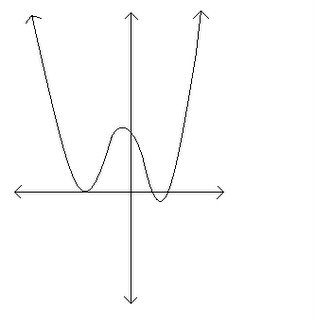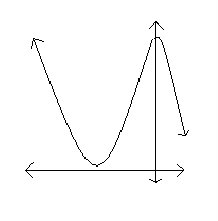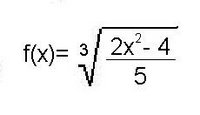## September 20, 2006

### Scribe Post: Day 9

Hey everyone, this is Suzanne. We started today’s class off with that quiz everyone had been looking forward to so very much. So I’ll start off by going over some of the methods Mr.K discussed for the first question, where we were asked to state how many roots the function had, and what the largest root was.

Unfortunately I don’t have the exact equation, so these graphs are an approximation. And not the greatest either. I'm no artist :pa) This function had two roots, but when you graph it on your calculator, it appears at first glance to have three roots. There are several ways to determine whether or not there is a root.

Method 1: First off, you can use the zero function on your calculator by pressing 2nd calc, zero. If the calculator comes up with a zero, then there’s a root there. But in this case, an error comes up saying ‘no sign change’. If this happens, then either there is no root, or the root touches the x-axis but does not cross it. This can be seen, for example, with the graph f(x)=x2.

Method 2: So we still need to figure out whether there is a root or not. We can use the zoom-in function on the calculator, closing in on the root until we can clearly see whether it touches the x-axis or not. (I don't know if you can really tell, but I promise you that it's not touching the x-axis in this diagram. Cross my heart.)Method 3: Finally, we can find the minimum. Press 2nd, calc, minimum. The procedure from there is the same as if you were calculating a zero. (First pick a point to the left, then to the right). If the minimum value is greater than zero, then there is definitely no root. I personally think this is the most efficient way to complete the problem.

b) This function was very sneaky… it appears at first to have three roots. However, by looking at the equation we can tell that there are actually 4 roots. The highest exponent is even, so we know that both outer arms of the graph must go in the same direction. In this case, there was a negative sign in front of the function, so we know they point downward. Therefore there must be another root somewhere off to the right. We can find this root two ways.

Method 1: Expand the size of the window until you see the root. Then it is just a case of using the zero function on your calculator.

Method 2: Go to the table by entering 2nd, table and find the zero. Well, in this case it’s a nice prettyful zero at 50, but of course we will not always be that lucky. Just look for where the y-values go from positive to negative, and you will have a good idea where to look for the zero. Then it’s just a case of using method 1 to find your root.

I'm not going to go into the rest of the quiz, since I don't have the questions. After the quiz we started to talk about inverses, which is going to lead into a lesson on logs tomorrow (I think). First of all we discussed that exponents are the inverse of logs. Mr. K said that logs of base 10 and e are the most frequently used, and that after high school they are pretty much the only thing we will see. So here are some of the ideas we went over:

1) Find the inverse of the function:We went over two ways of doing this.

Method 1: List step-by-step what is happening to x, then go through those steps from last to first, undoing whatever that step did.

Method 2) Switch x with y in the equation and then isolate y. You'll get the same answer as stated above. Note that this method won’t work on all functions. For example, f(x)= (2x-1)/(x+3) will not work. The reason for this is that 2 things are happening to x at once.

2) We have 2 functions: f(x)=2x+3 and g(x)= ½(x-3). What is f(g(x))?

f(g(x))= 2(1/2(x-3)) +3
= x-3+3
=x

In this case what you put in is what you get out. In other words, these two functions are inverses.

And that’s all I have guys. Stay tuned for tomorrow’s thrilling continuation! And the lucky winner of the ‘next scribe’ competition is…. Jann!

Anh said...

hey suzanne,
your scribe post is soo neat and really appealing to look at. Yours graphs are perfect. You did a really good job =)

christian said...

YEA! I love suzanne's explanations (when we're on an agreement)! So concise.. so easy to understand. I bet my pet can read your post and do our exercises. You have to tell me how you did your graphs. Paint isn't my forte.Anonymous said...

suzanne, i thought your graphs were very nicely done. and i also thought that your post was easy to follow. i noticed that you tried to make a square where it says f(x)=x [sup]2[/sup], and it didn't come out in the post. instead of using square brackets for that code replace it with these kinda brackets < > and it should work. if i'm not making sense, i can explain to you in class. anyway just thought i'd help you out with that... but otherwise good job =)

Suzanne said...

Thank you linger :) I was wondering why it wasn't working..

♫ Jann ♫ said...

Hi Suzanne,
First i want to thank you for picking me for the next scribe (^_^). I have no homework today thats why. Hehe. Anyways, your scribe is very organized and well done. Thanks for adding the details on the quiz we had.